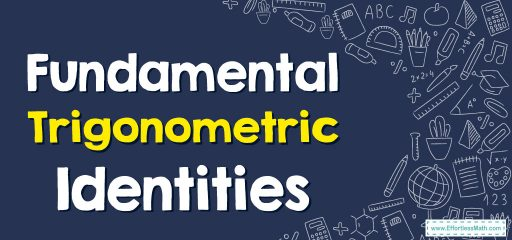# Fundamental Trigonometric Identities

Trigonometric identities are equations that relate various trigonometric functions and are true for any variable value in the domain. In this post, you can learn fundamental trigonometric identities.## A step-by-step guide tofundamental trigonometric identities

The basic trigonometric identities or fundamental trigonometric identities are those trigonometric functions that are true every time for the variables.

The following equations are eight of the most basic and important trigonometric identities. These equations are true for any angle. Countless additional identities can be formed from them. These eight things should be kept in mind.

• $$\color{blue}{cot\left(θ\right)=\frac{cos\:\left(\theta \right)}{sin\:\left(\theta \right)}}$$
• $$\color{blue}{tan⁡\:\left(\theta \right)=\frac{sin\:\left(\theta \right)}{cos\:\left(\theta \right)}}$$
• $$\color{blue}{cot\left(θ\right)=\frac{1}{tan\:\left(\theta \right)}}$$
• $$\color{blue}{sec\left(θ\right)=\frac{1}{cos\:\left(\theta \right)}}$$
• $$\color{blue}{csc\left(θ\right)=\frac{1}{sin\:\left(\theta \right)}}$$
• $$\color{blue}{\left(sin\left(θ\right)\right)^2+\left(cos\left(θ\right)\right)^2=1}$$
• $$\color{blue}{1+\left(tan\left(θ\right)\right)^2=\left(sec\left(θ\right)\right)^2\:\:}$$
• $$\color{blue}{1+\left(cot\left(θ\right)\right)^2=\left(csc\left(θ\right)\right)^2}$$

### What people say about "Fundamental Trigonometric Identities - Effortless Math: We Help Students Learn to LOVE Mathematics"?

No one replied yet.

X
30% OFF

Limited time only!

Save Over 30%

SAVE $5 It was$16.99 now it is \$11.99